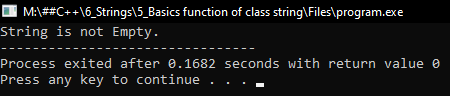# Functions of String Class in C++

## Functions of String Class in C++ with Examples:

In this article, I am going to discuss the basic Functions of the String Class in C++ Language with examples. Please read our previous article, where we discussed String Class in C++ with examples i.e. how to create the object of a string class.

##### Basic Functions of String Class in C++

Now let’s see the built-in functions of the String class in C++.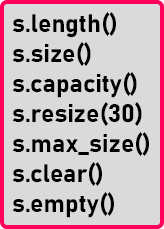Here we have a few basic functions of the string class in C++. Let us discuss all these functions one by one with examples.

##### length() function of String Class in C++:

This function will find out the length of the string. So how to call this function? We will create an object of string class and then we need to call the length function as follows.

String str1 = “Hello”;
str1.length();

Here we have created and initialized the object of type string. Next, we will call the length function through the string object as ‘str1.length()’. This will find out the length of this string. So, the functions are called upon the object because they are member functions. We have to use the dot operator for calling the member functions. Suppose we have one more string as

String str1 = “Welcome”;
str2.length();

Now for finding the length of this string we can call the function ‘str2.length()’. Depending on the object the function will be called. So, the benefit of object orientation is we can call the functions upon object since they are inside the object. It means ‘str1’ is having a string ‘hello’ as well as all the various functions like length and all the above-mentioned functions. Now let us go to the next function.

##### size() function of String Class in C++:

This is also the same thing. We can call length or we can call size. Both have the same meaning.

##### Program for length() and size() functions of String Class in C++
```#include <iostream>
#include <string>
using namespace std;
int main()
{
string str = "Hello World";
cout << "Length: " << str.length () << endl;
cout << "Size: " << str.size () << endl;
}
```
###### Output: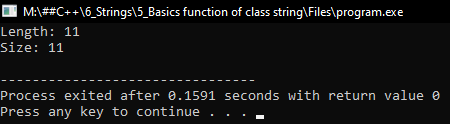##### capacity() function of String Class in C++:

This is different in size and length. This gives the capacity of a string. As we have already explained in the previous article that it will not create an array exactly of the given string size, it will create a little bigger size. So, it will give the capacity of this string. If we gave a bigger string or larger string it will increase the capacity and also the length of the string. It is dynamic it will be changing its size.

##### Program for capacity() function of String Class in C++:
```#include <iostream>
#include <string>
using namespace std;
int main()
{
string str = "Hello World";
cout << "Capacity: " << str.capacity () << endl;
}
```
###### Output: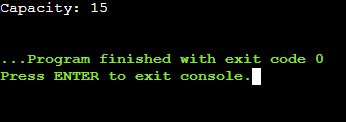##### resize() function of String Class in C++:

This function is used to increase the capacity of a string. We can mention the size and change the capacity.

##### Program for resize() function of String Class in C++
```#include <iostream>
#include <string>
using namespace std;
int main()
{
string str = "Hello World";
str.resize (40);
cout << "Capacity: " << str.capacity () << endl;
}
```
###### Output: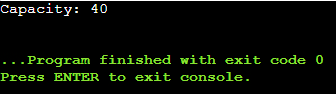##### max_size() function of String Class in C++:

Depending on the compiler this function gives the possible maximum size for a string we can have in the compiler. This may vary from compiler to compiler. So, this will give you the maximum possible size like thousand characters or lacs of characters. By this function, we can know the size.

##### Program for max_size() function of String Class in C++:
```#include <iostream>
#include <string>
using namespace std;
int main()
{
string str = "Hello World";
cout << "Max Size: " << str.max_size () << endl;
}
```
###### Output: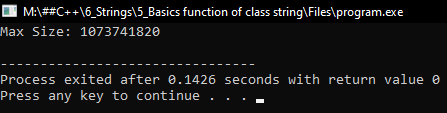##### clear() function of String Class in C++:

This will clear the contents of the string.

##### Program for clear() function of String Class in C++:
```#include <iostream>
#include <string>
using namespace std;
int main()
{
string str = "Hello World";
str.clear ();
cout << "Length: " << str.length () << endl;
}
```
###### Output: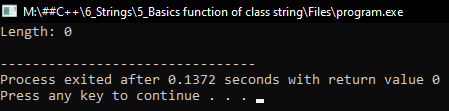##### empty() function of String Class in C++:

This will find out whether a string is empty or not.

##### Program for empty() function of String Class in C++:
```#include <iostream>
#include <string>
using namespace std;
int main()
{
string str = "Hello World";
if (!str.empty ())
{
cout << "String is not Empty.";
}
}
```
###### Output: